# Week 5: Transformers

[reveal]

Abstract:

This lecture will introduce transformers.

## Plan for the Day

• Attention, Attention!
• Transformers: Attention is All You Need.
• Transformer extensions:
• Reformers - alternative attention operator
• Linformers - linear complexity
• Transformer training difficulty
• Switch Transformers
• Going beyond NLP
• Image is Worth 16x16 Words
• AlphaFold 2

## Think back to last week: Seq2Seq

• Encoder compresses inut into a number of hidden notes - the state vector.
• Decoder , initialized with the context vector, generates the output (translation)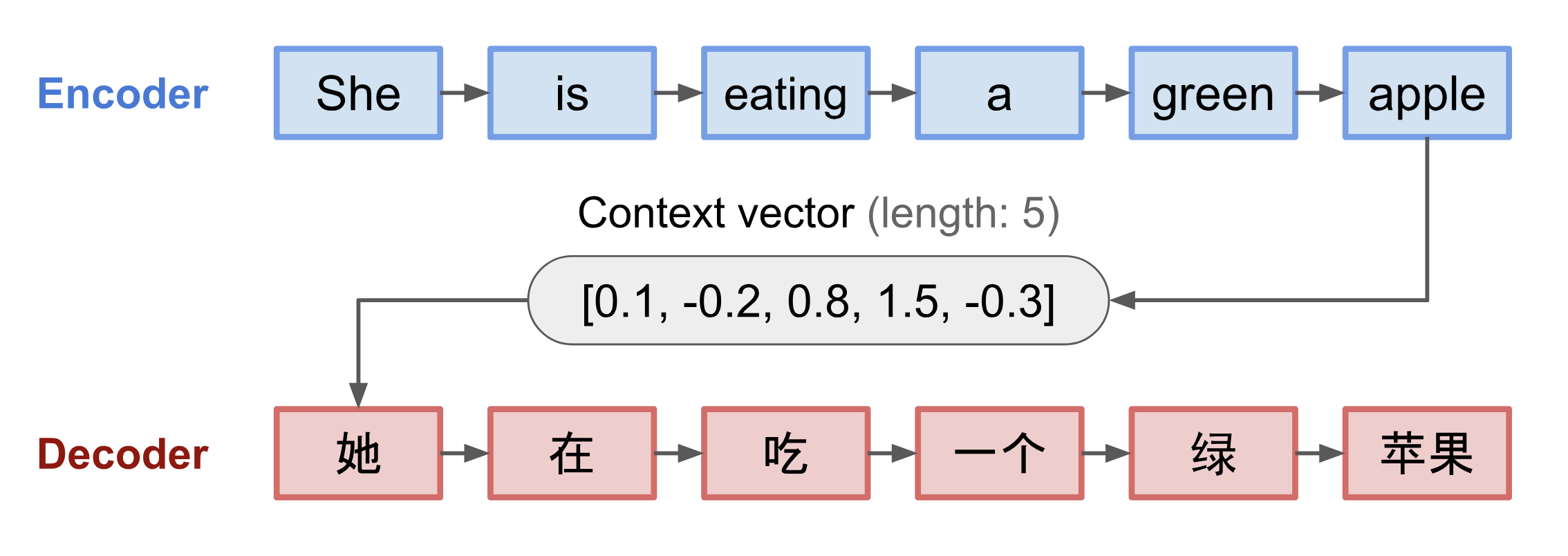• Memory, and gradient stability, remain the key limitation even when LSTMs and GRUs are used.

## Neural Machine Translation by Jointly Learning to Align and Translate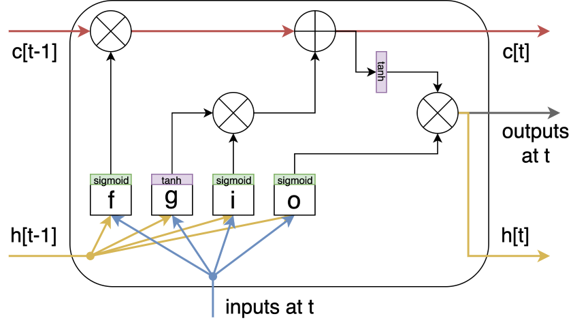## Learning Alignment - the first Attention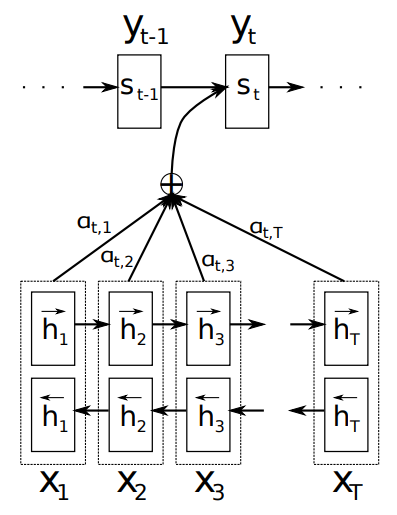• Fixed-length context vector was a key performance bottleneck in 2015.
• Bahdanau et al. (2015) propose to (soft-)search for parts of a source
sentence that are relevant to predicting a target word.
• The method, based on the Cho et al. (2014) Encoder–Decoder:
• Encoder: bi-directional RNN.
• Decoder: gated RNN.
• Innovation: each time-step gets its own separate context vector: $\large c_t = \sum_{j=1}^T ( \alpha_{t,j} h_t)$ $\large \alpha_{t,j} = \frac{\exp(e_{t,j})} {\sum_{k=1}^T\exp(e_{t,k})}$ $\large e_{t,j} = f(s_{t-1}, h_j)$

Function $f$ implemented as a fully connected layer.

## Attention, Attention!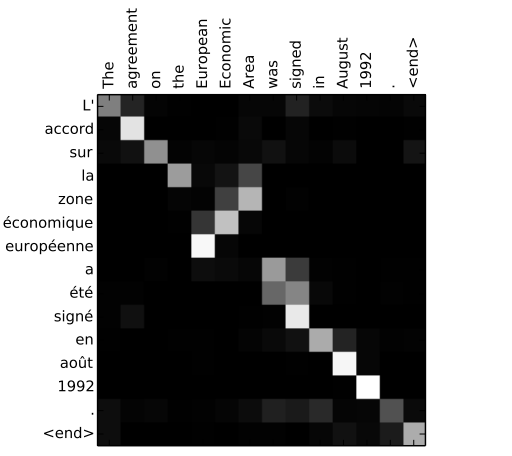## Plan for the Day

• Attention, Attention!
• Transformers: Attention is All You Need.
• Transformer extensions:
• Reformers - alternative attention operator
• Linformers - linear complexity
• Transformer training difficulty
• Switch Transformers
• Going beyond NLP
• Image is Worth 16x16 Words
• AlphaFold 2

## Attention is All You Need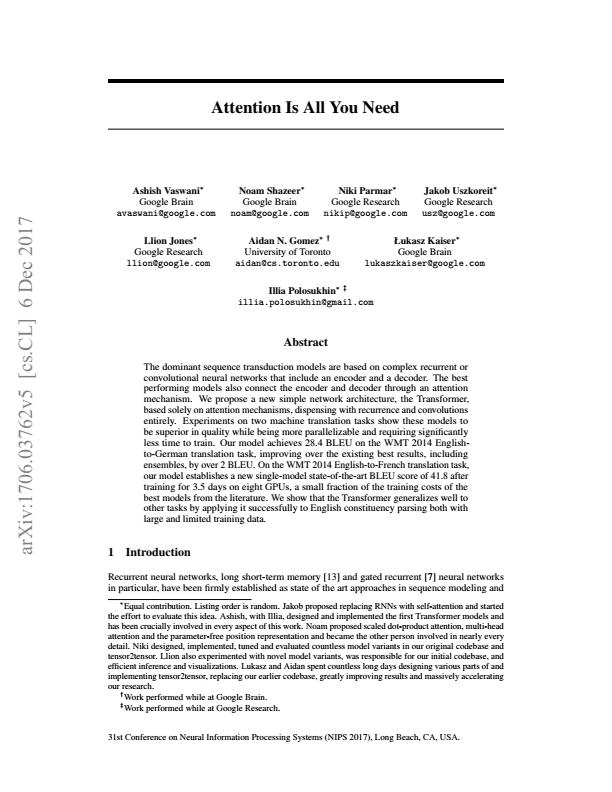## Attention is All You Need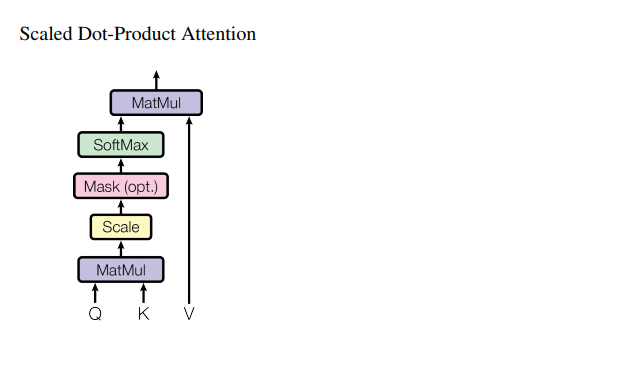• Scaled dot-product attention (no parameters):

$\text{attention}(Q,K,V)=\text{softmax}\left(\frac{QK^{\top}}{\sqrt{d_{K}}}\right) V$

## Attention is All You Need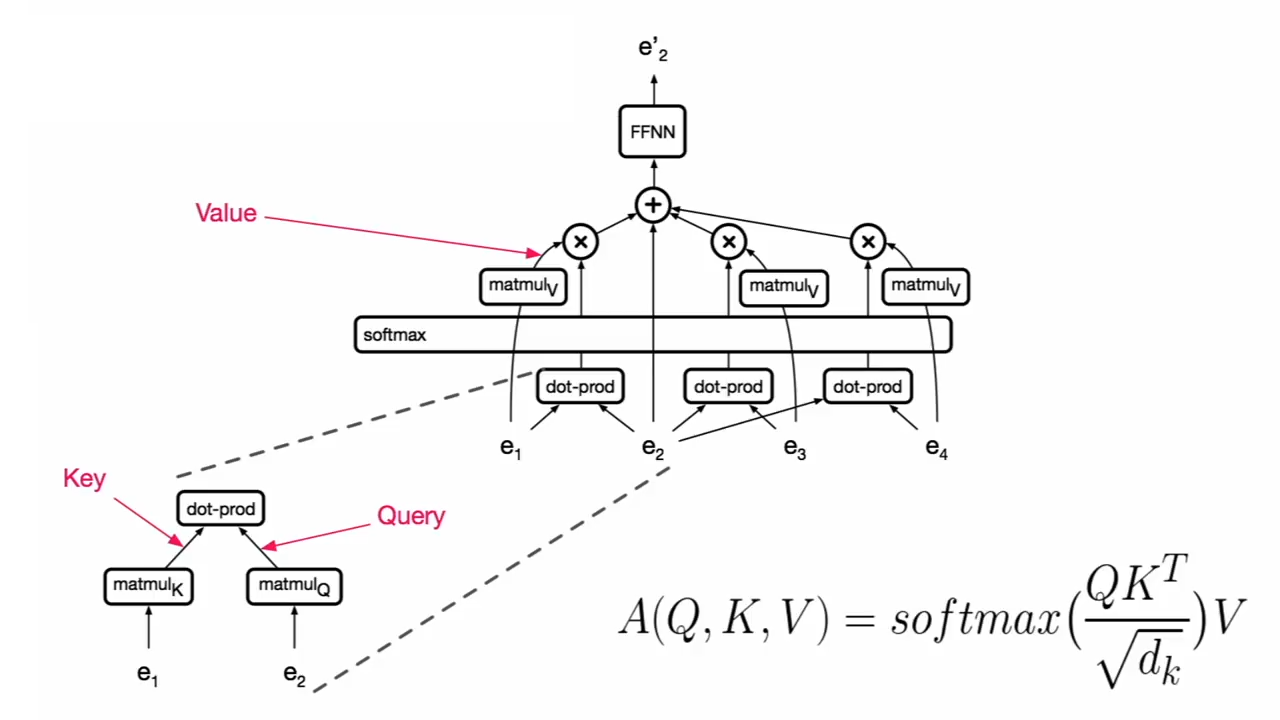## Attention is All You Need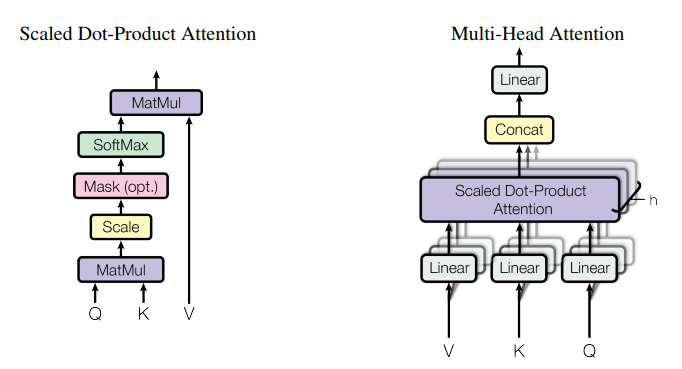• Scaled dot-product attention (no parameters):

$\text{attention}(Q,K,V)=\text{softmax}\left(\frac{QK^{\top}}{\sqrt{d_{K}}}\right) V$

• Multi-head attention (parameters: $W_0, W_1^Q, W_1^K, W_1^V, \dots$): $\text{MultiHead}(Q,K,V)=\text{concat}(\text{head}_{1},\dots,\text{head}_{h}) W_{0}$ $\text{head}_i=\text{attention}(QW_i^Q, KW_i^K, VW_i^V)$

## Attention is All You Need: the Transformer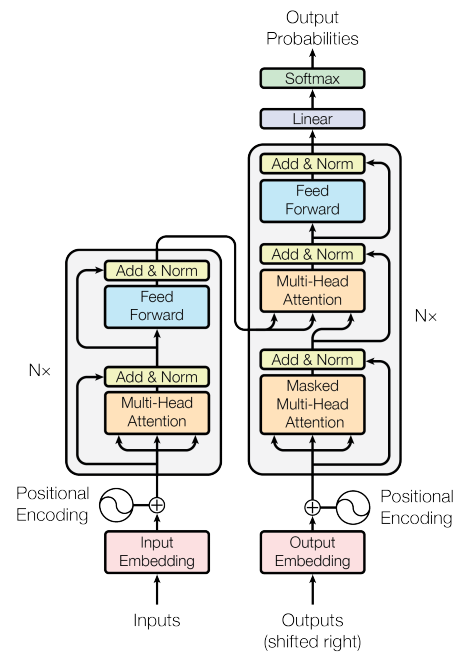## Attention is All You Need

The Transformer achieves better BLEU scores than previous state-of-the-art models on the English-to-German
and English-to-French newstest 2014 tests at a fraction of the training cost.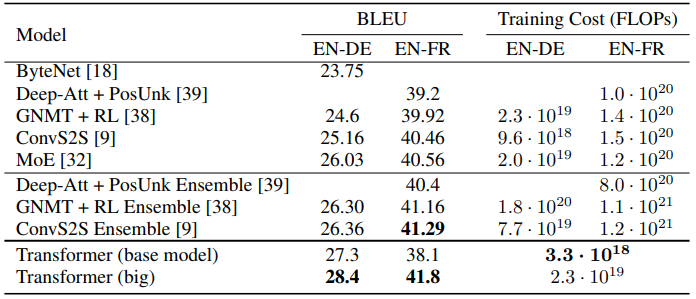## Plan for the Day

• Attention, Attention!
• Transformers: Attention is All You Need.
• Transformer extensions:
• Reformers - alternative attention operator
• Linformers - linear complexity
• Transformer training difficulty
• Switch Transformers
• Going beyond NLP
• Image is Worth 16x16 Words
• AlphaFold 2

## Reformers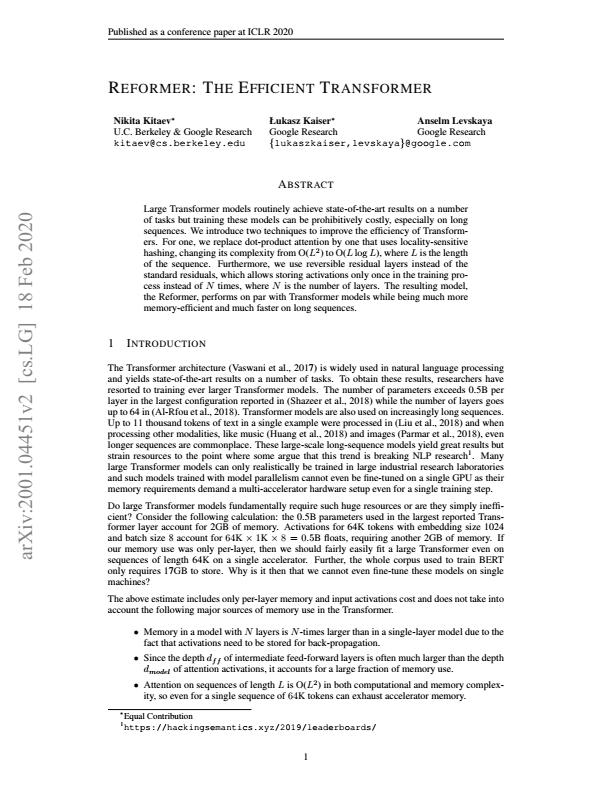## Reformers: Locality Sensitive Hashing

Rather than attending all-to-all, split the sequence up. Kitaev et al. (2020) employ a hashing scheme first proposed by Andoni et al. (2015).## Reformers performance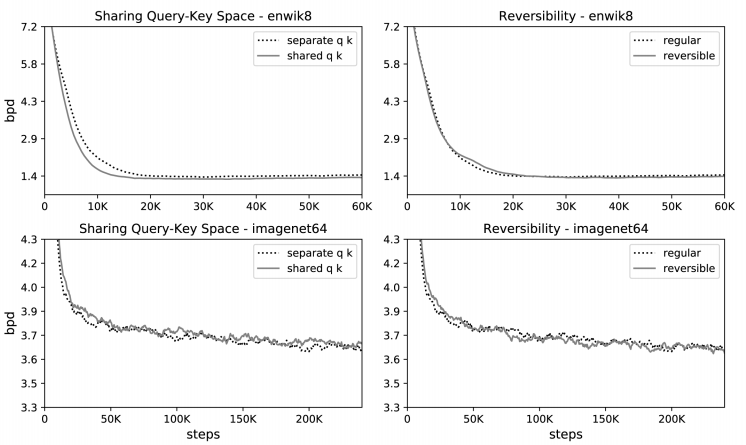Loss: log-likelihood and perplexity expressed in bits per dimension.

## Linformers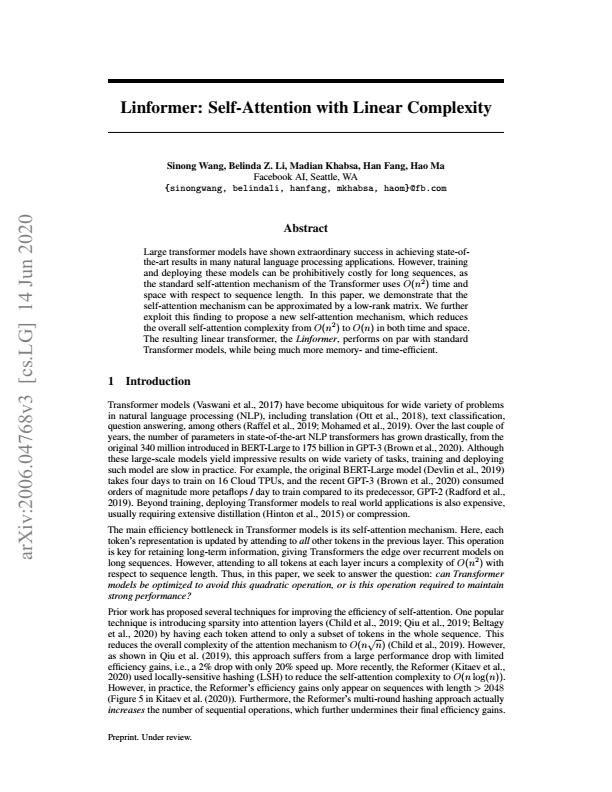## Linformers

• Basic attention (no parameters):

$\text{attention}(Q,K,V)=\underbrace{\text{softmax}\left(\frac{QK^{\top}}{\sqrt{d_{K}}}\right)}_\text{P} V$

• Wang et al. prove theoretically and check empirically that P is low rank.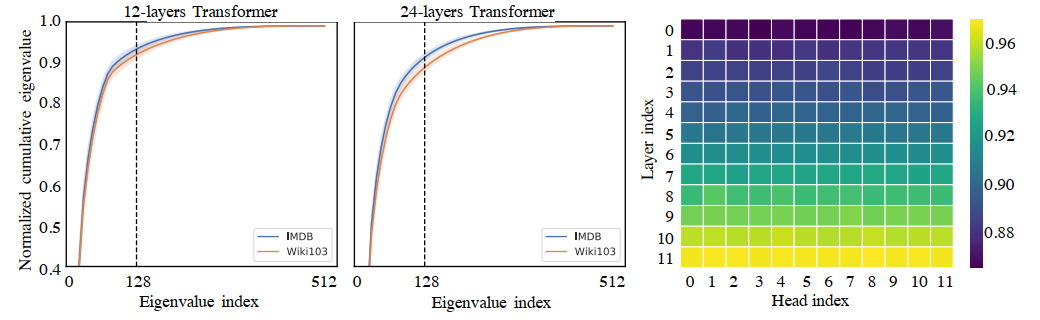## Linformers

The idea is very simple - add a simple projection between the weighted K, Q and their joint dot product.
This fixes to a constant the dimension of the matrices entering the self-attention mechanism.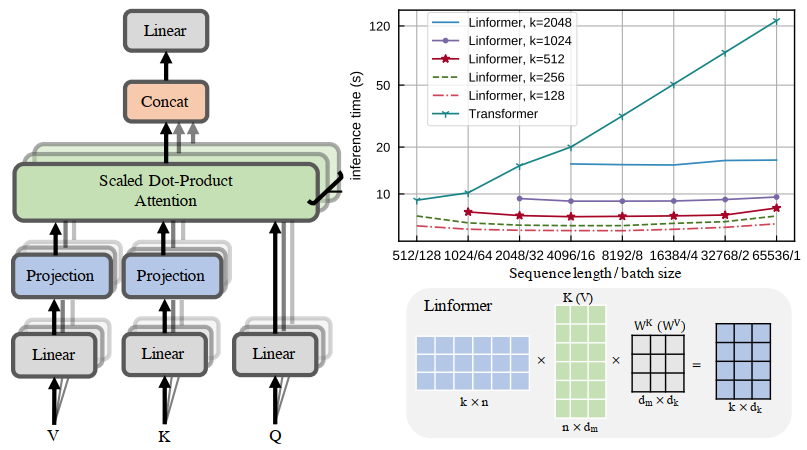## Linformers performance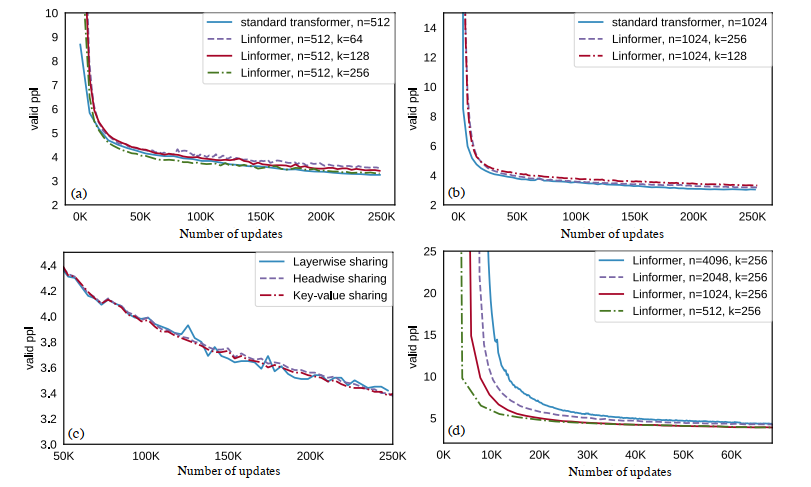## Training Transformers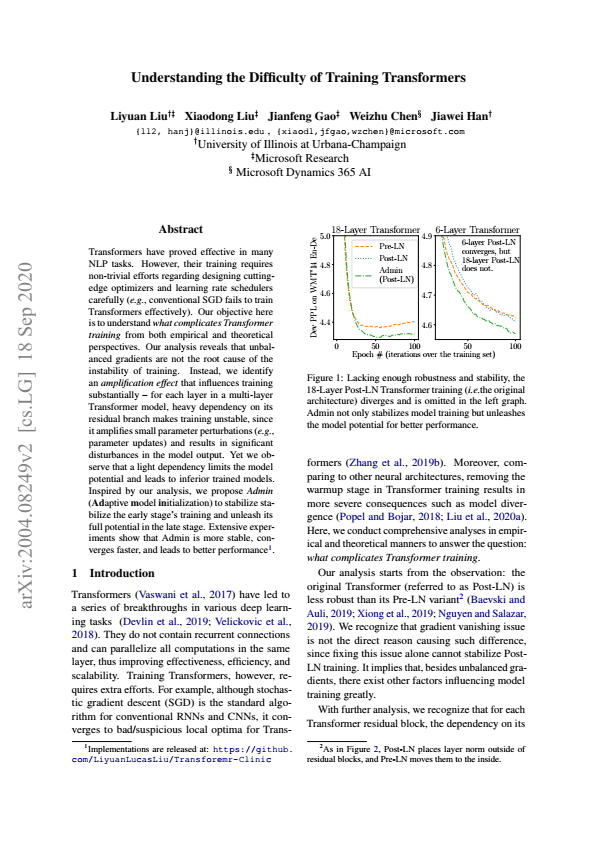## Layer Normalization in Transformers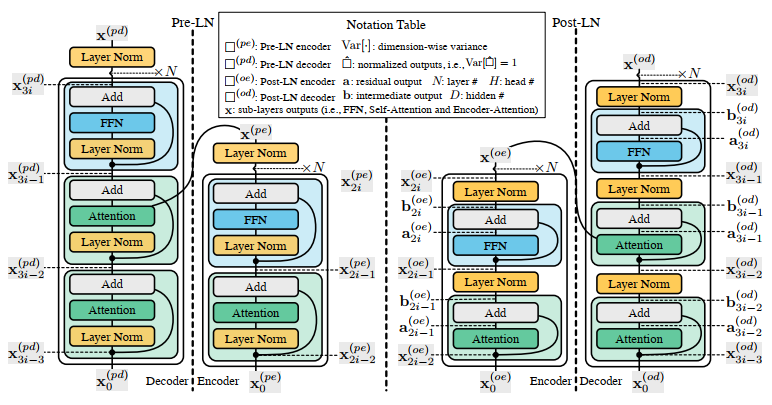## Training Transformers

Amplification-induced Instability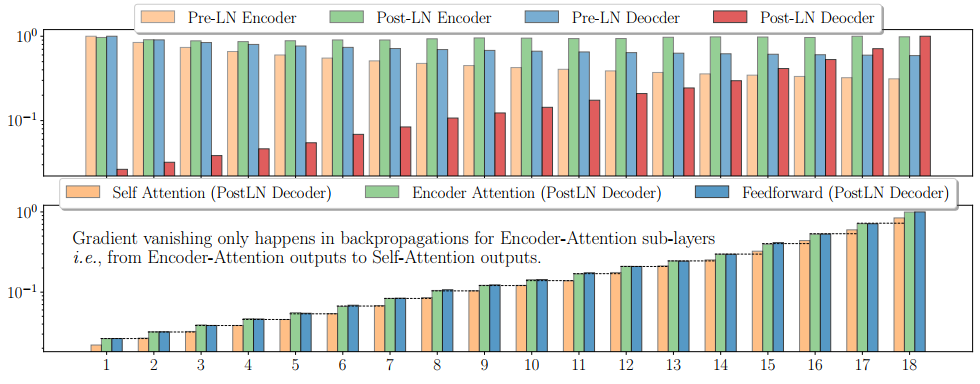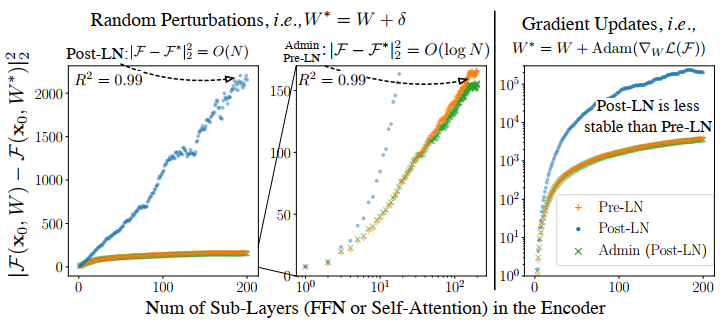## Switch Transformers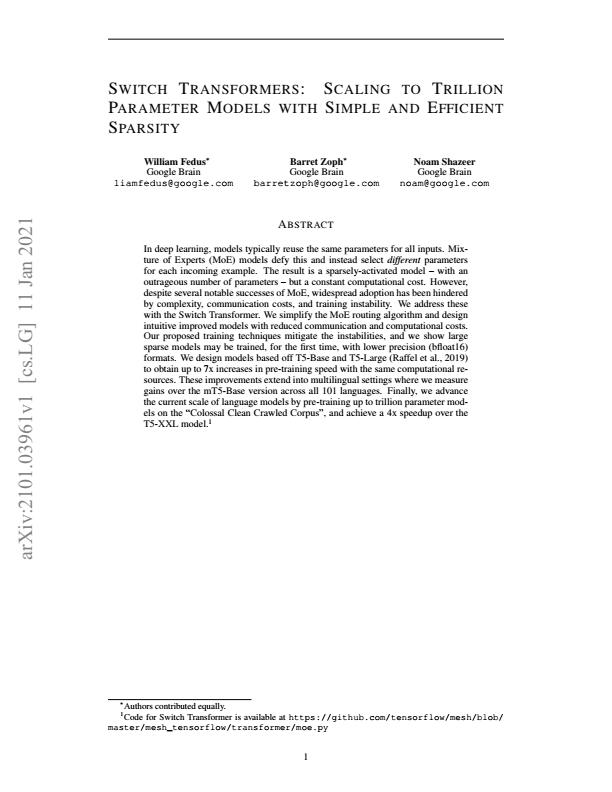## Switch Transformers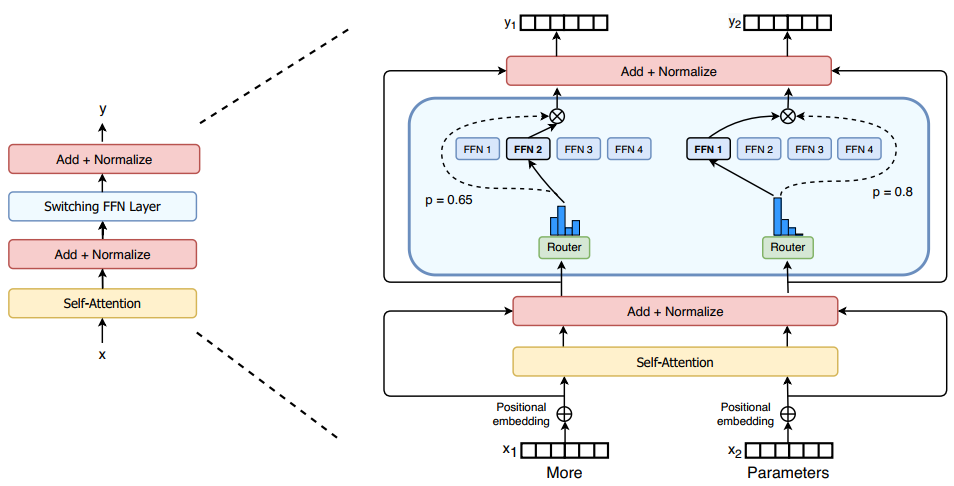## Switch Transformers - speed-up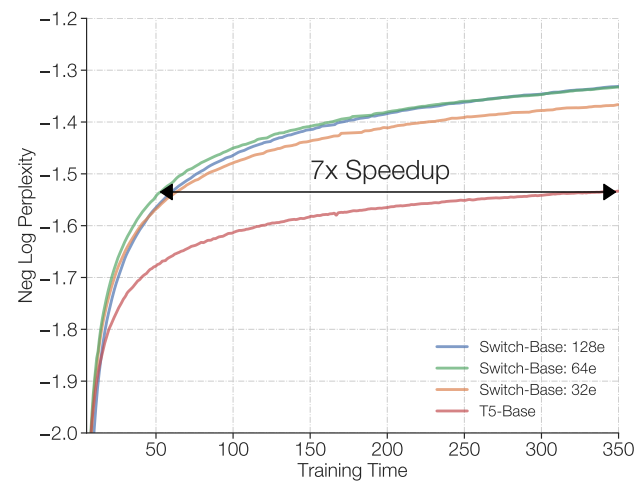## Switch Transformers - multi-task performance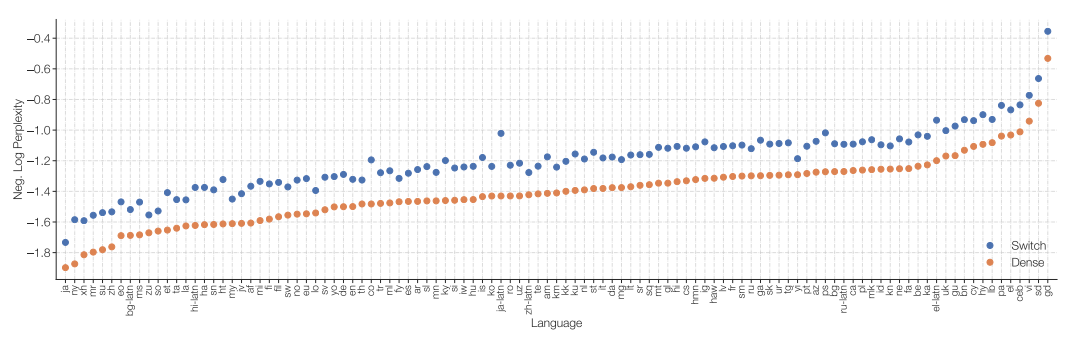## Plan for the Day

• Attention, Attention!
• Transformers: Attention is All You Need.
• Transformer extensions:
• Reformers - alternative attention operator
• Linformers - linear complexity
• Transformer training difficulty
• Switch Transformers
• Going beyond NLP
• Image is Worth 16x16 Words
• AlphaFold 2

## Image is Worth 16x16 Words Embedding and Architecture## Image is Worth 16x16 Words## Image is Worth 16x16 Words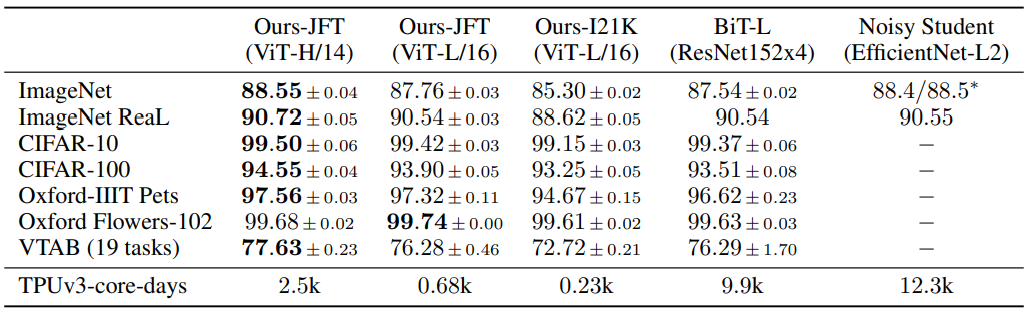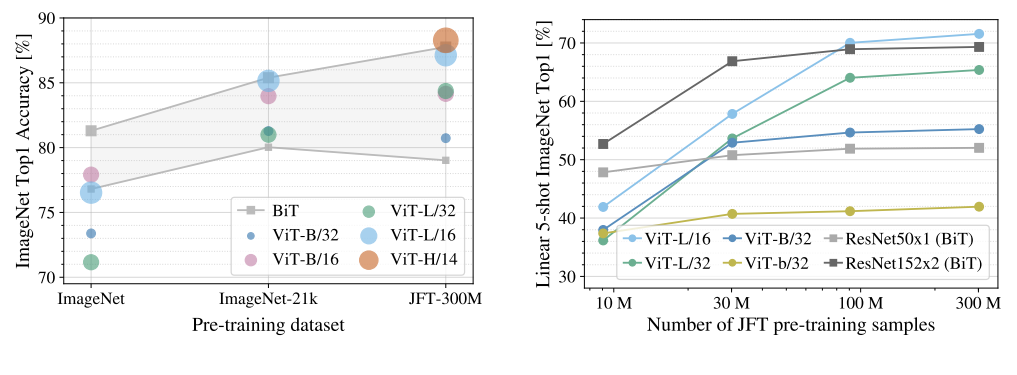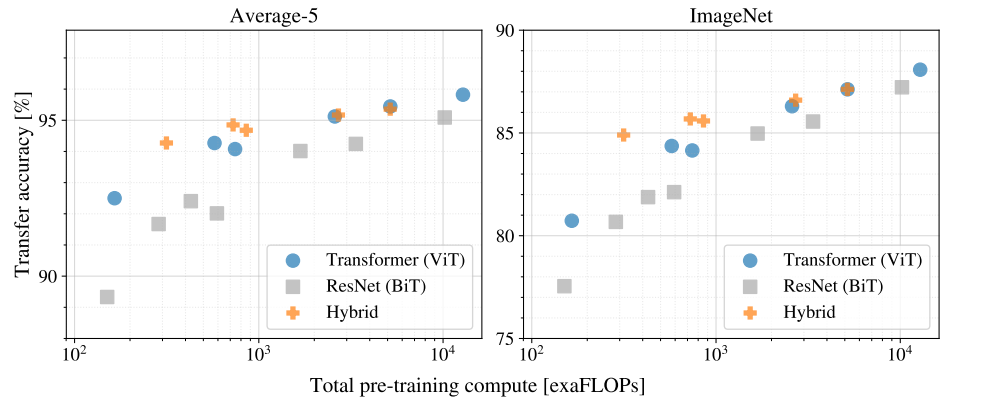## AlphaFold - the protein folding problem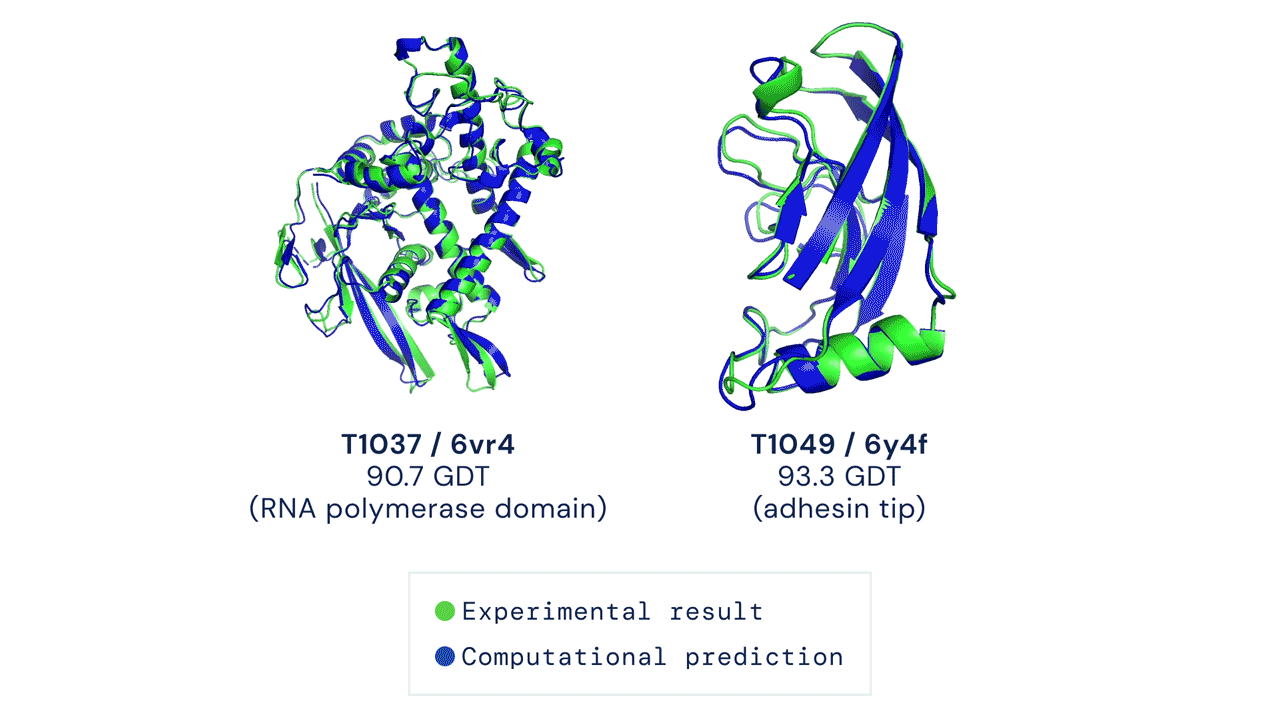## Convolutional solution: AlphaFold 1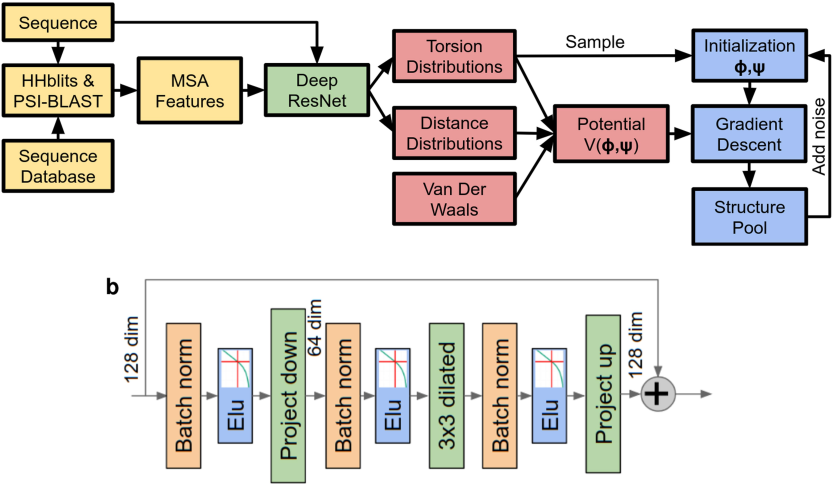## Transformer-based solution: AlphaFold 2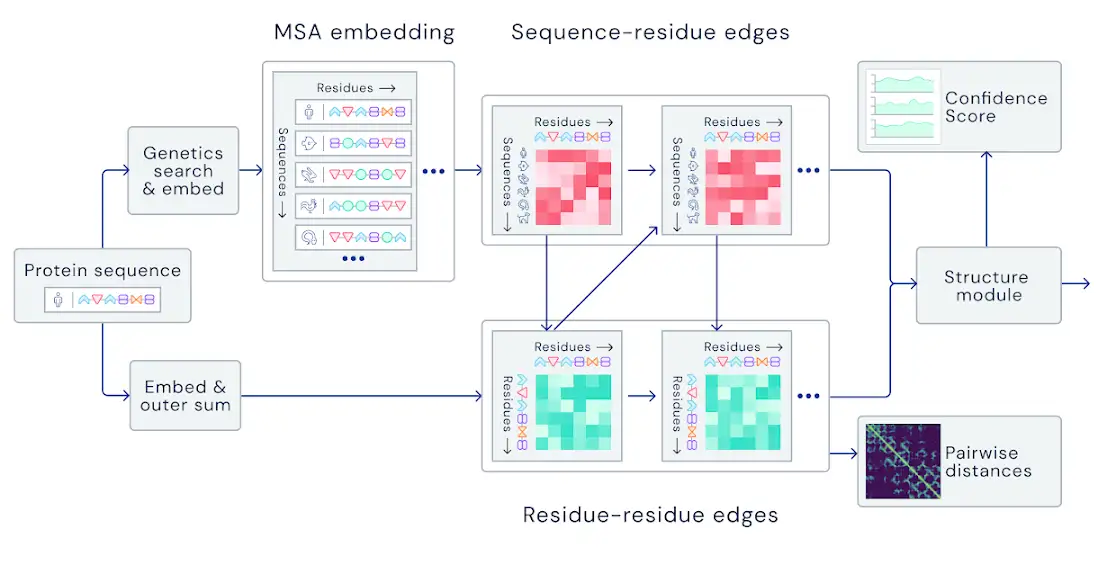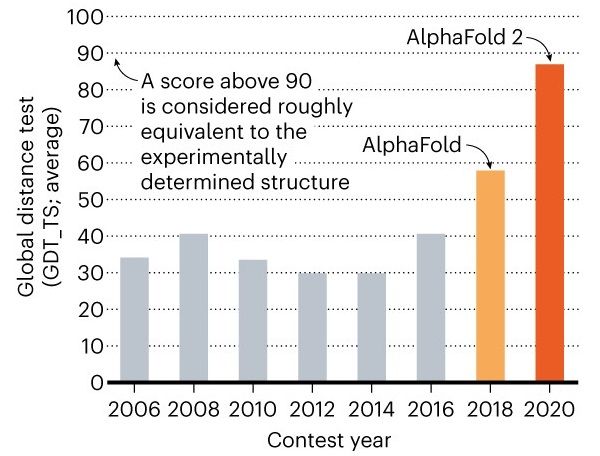## Summary of the Day

• Attention, Attention!
• Transformers: Attention is All You Need.
• Transformer extensions:
• Reformers - alternative attention operator
• Linformers - linear complexity
• Transformer training difficulty
• Switch Transformers
• Going beyond NLP
• Image is Worth 16x16 Words
• AlphaFold 2